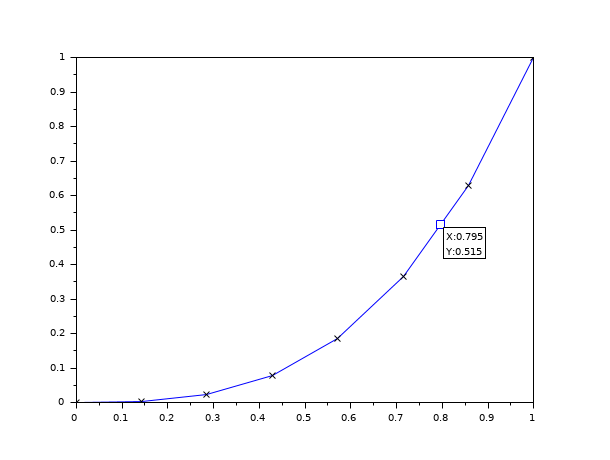Change language to:
Français - 日本語 - Português - Русский

See the recommended documentation of this function

Scilab Help >> Graphics > Datatips > datatipSetInterp

# datatipSetInterp

Set the interpolation mode for datatip.

### Syntax

`datatipSetInterp(datatip_handle,interp_mode)`

### Arguments

datatip_handle

A handle of the tip.

interp_mode

A boolean, if false the tips can only be located at the mesh points, if true interpolation is made between mesh points. The default value is true.

### Description

Set the interpolation mode for tip location.

### Examples

```x=linspace(0,1,8)';
y=x.^3;
clf()
plot(x,y);
e=gce();p=e.children(1);//get the handle on the polyline
p.mark_mode="on";p.mark_style=2;p.mark_size=5;
t=datatipCreate(p,[0.8,0.512]);
datatipSetInterp(t,%f);//now move the datatip```